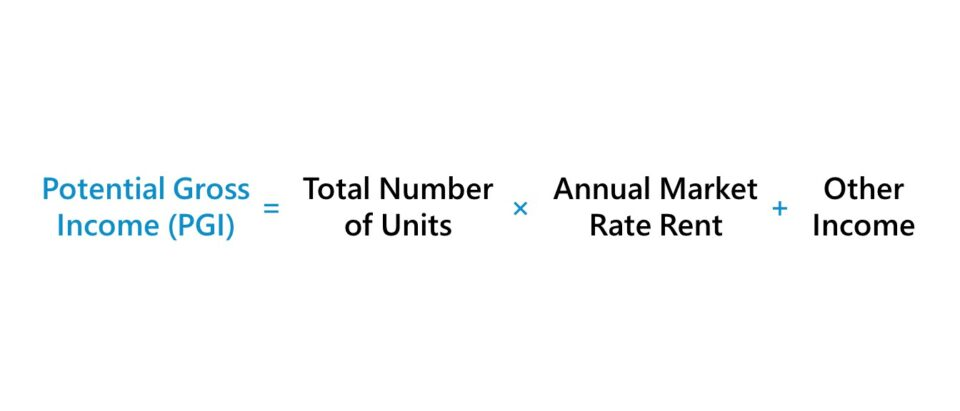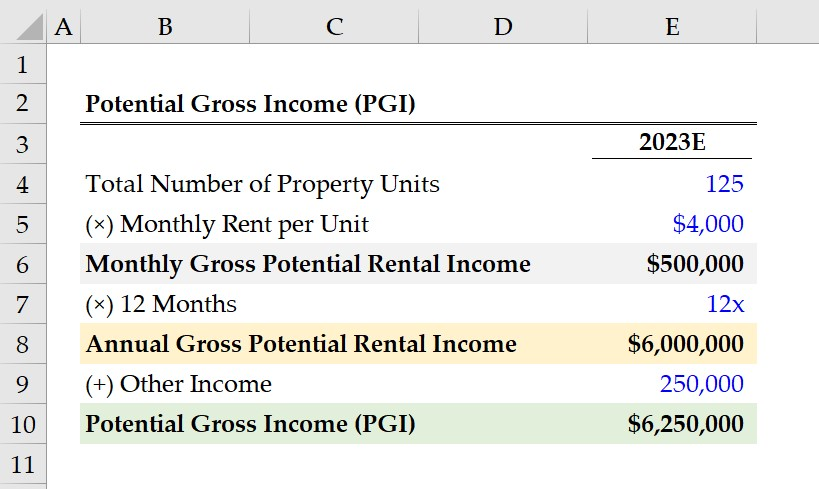Welcome to Wall Street Prep! Use code at checkout for 15% off.# Potential Gross Income (PGI)

Guide to Understanding the Potential Gross Income (PGI)## How to Calculate Potential Gross Income?

The potential gross income (PGI), or “gross potential income”, is a real estate investing metric that estimates the maximum income that could be derived from a rental property.

The potential gross income is a pro-forma metric with two implicit assumptions, which are an occupancy rate of 100% and on-time rent collection.

• Full Occupancy Rate → A property with full occupancy has no vacant units, i.e. a vacancy rate of 0%. Each unit available for rent is assumed to be occupied by a tenant and generates income for the property owner.
• On-Time Rent Collection → If the collection of rent payments is on-time, all tenants are assumed to have fulfilled their payment obligations per the contractual rental agreement. The income requirements and credit checks performed on potential tenants are intended to mitigate the risk of a tenant being late on rent payments or defaulting on the lease arrangement, resulting in eviction and property foreclosure.

The potential gross income is the hypothetical total income that can be received by the property owner if all units are fully occupied at the current market rate, and if there are no issues collecting rent payments from tenants.

Therefore, the potential gross income (PGI) matters to real estate investors because it represents the total earnings potential of a rental property investment.

The other sources of income – aside from rental income – must also be included in the calculation, such as the fees charged to tenants for use of on-premise amenities, laundry or dry cleaning services, parking permits, storage units, and pet fees.## Potential Gross Income vs. Effective Gross Income: What is the Difference?

Under the specific pretense of underwriting in real estate, the income of a property is separated into two categories:

• Potential Gross Income (PGI) → The potential gross income is the maximum on the total earnings that a rental property can generate (i.e. the “ceiling”), assuming no vacancy or credit losses.
• Effective Gross Income (EGI) → In contrast, the effective gross income is the approximate income that a rental property can produce upon adjusting for vacancies and credit (collection) losses.

The potential gross income (PGI) is the starting point to calculate the effective gross income (EGI), the estimated income that a property can generate after factoring in the costs incurred related to vacancy and credit losses.

Since effective gross income (EGI) deducts the costs attributable to vacancy and credit losses, the EGI will be the lesser of the two.

## Potential Gross Income Formula (PGI)

The formula to calculate the potential gross income is as follows.

Potential Gross Income (PGI) = (Total Number of Units × Annual Market Rate Rent) + Other Income

Where:

• Total Number of Units: The total number of units in the property that are available for rent and can generate income on behalf of the property owner.
• Annual Market Rate Rent: The monthly rent at the current market rate, converted to an annualized figure.
• Other Income: The on-premise services and other side sources of income that a property can generate (e.g. laundry fees, amenities access).

Of course, a full occupancy rate and no collection losses is an unrealistic set of assumptions; hence the potential gross income is then adjusted to determine the effective gross income.

Effective Gross Income (EGI) = Potential Gross Income (PGI)  Vacancy and Credit Losses

## Potential Gross Income Calculator

We’ll now move on to a modeling exercise, which you can access by filling out the form below.#### Get the Excel Template!Submitting...

## 1. Real Estate Rental Property Assumptions

Suppose a real estate property owner is estimating the potential gross income (PGI) of a rental property investment in 2023.

There are 125 units available for rent in the residential building, while the monthly rent per unit at the current market rate is \$4k each month.

• Total Number of Units = 125 Units
• Monthly Rent per Unit, Market Rate = \$4,000

The product of the property’s total number of units and market-rate rent is the gross potential rental income that the property can generate on a monthly basis, which comes out to \$500k.

• Monthly Gross Potential Rental Income = 125 Units × \$4,000 = \$500,000

Our next step is to convert the monthly gross potential rental income of \$500k into an annualized figure, which we’ll determine by multiplying by 12 to arrive at \$6 million as the annual gross potential rental income.

• Annual Gross Potential Rental Income = \$500,000 × 12 Months = \$6,000,000

Because of the full occupancy rate and on-time rent collection assumptions, there is no need for any adjustments pertaining to vacancy and credit losses.

## 2. Potential Gross Income Calculation Example (PGI)

In the final step, we must add any other sources of income to our gross potential rental income, which we’ll assume is \$250,000 for the entire year.

Said differently, around \$167 is extracted from the property’s tenants per month on top of rent.

• Other Income = \$250,000

In conclusion, the sum of the annual gross rental income and other income amounts to \$6.25 million, which represents the rental property’s potential gross income (PGI) in 2023.

• Potential Gross Income (PGI) = \$6,000,0000 + \$250,000 = \$6,250,00020+ Hours of Online Video Training

### Master Real Estate Financial Modeling

This program breaks down everything you need to build and interpret real estate finance models. Used at the world's leading real estate private equity firms and academic institutions.

Inline Feedbacks